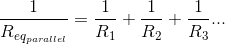# Parallel Resistors

## Working principles

• Voltage across a resistor generates a current.
• Current flows through the path of the least resistance.
• Resistors can be combined in series and parallel configurations.

### Applicable formulasFor two resistors in parallel with different values:If two resistances or impedances in parallel are of the same value, their equivalent resistance is half the value of one resistor, or R/2. For three equivalent resistors in parallel: R/3, and so on.

### Experiment

• Calculate the equivalent impedance for the combined resistors. What will be the total current shared by the resistor network? What will be the current in each resistor branch?